# How to build Machine Learning Apps with Streamlit?In this article, I’m going to show some interesting features about Streamlit, building an app with the purpose of inspecting data and build Machine Learning model on them.

Streamlit is an open-source Python library that makes it easy to build beautiful apps for machine learning. You can easily install it via pip in your terminal and then start writing your web app in Python.

In this article, I’m going to show some interesting features about Streamlit, building an app with the purpose of inspecting data and build ML model on them. To do so, I will use the very basic Iris dataset and perform some classifications on it. However, if you are interested in more advanced potentialities of this tool, I suggest you read this tutorial.

Having said that, let’s start building our app. I will write all my code in one file, called iris.py, so that I will be able to run it from my terminal via streamlit iris.py.

In the end, the full code of my app will be the following:

``````import streamlit as st
import pandas as pd
import numpy as np
import plotly.express as px
import seaborn as sns
import matplotlib.pyplot as plt
import plotly.graph_objects as gost.title('Iris')df = pd.read_csv("iris.csv")if st.checkbox('Show dataframe'):
st.write(df)st.subheader('Scatter plot')species = st.multiselect('Show iris per variety?', df['variety'].unique())
col1 = st.selectbox('Which feature on x?', df.columns[0:4])
col2 = st.selectbox('Which feature on y?', df.columns[0:4])new_df = df[(df['variety'].isin(species))]
st.write(new_df)
# create figure using plotly express
fig = px.scatter(new_df, x =col1,y=col2, color='variety')
# Plot!st.plotly_chart(fig)st.subheader('Histogram')feature = st.selectbox('Which feature?', df.columns[0:4])
# Filter dataframe
new_df2 = df[(df['variety'].isin(species))][feature]
fig2 = px.histogram(new_df, x=feature, color="variety", marginal="rug")
st.plotly_chart(fig2)st.subheader('Machine Learning models')from sklearn.model_selection import train_test_split
from sklearn.tree import DecisionTreeClassifier
from sklearn.metrics import confusion_matrix
from sklearn.svm import SVCfeatures= df[['sepal.length', 'sepal.width', 'petal.length', 'petal.width']].values
labels = df['variety'].valuesX_train,X_test, y_train, y_test = train_test_split(features, labels, train_size=0.7, random_state=1)alg = ['Decision Tree', 'Support Vector Machine']
classifier = st.selectbox('Which algorithm?', alg)
if classifier=='Decision Tree':
dtc = DecisionTreeClassifier()
dtc.fit(X_train, y_train)
acc = dtc.score(X_test, y_test)
st.write('Accuracy: ', acc)
pred_dtc = dtc.predict(X_test)
cm_dtc=confusion_matrix(y_test,pred_dtc)
st.write('Confusion matrix: ', cm_dtc)elif classifier == 'Support Vector Machine':
svm=SVC()
svm.fit(X_train, y_train)
acc = svm.score(X_test, y_test)
st.write('Accuracy: ', acc)
pred_svm = svm.predict(X_test)
cm=confusion_matrix(y_test,pred_svm)
st.write('Confusion matrix: ', cm)``````

Now, let’s examine each piece of code. As first thing, once imported the needed packages, I want to set my app’s title and import my data:

``````import streamlit as st
import pandas as pd
import numpy as np
import plotly.express as px
import seaborn as sns
import matplotlib.pyplot as plt
import plotly.graph_objects as gost.title('Iris')df = pd.read_csv("iris.csv")``````

Now I want a first option which allows the user to decide whether or not to show the entire dataset. I can do this (and many other interaction widgets) with the following syntax:

``````if st.checkbox('Show dataframe'):
st.write(df)``````

Even though naive, we can already launch our baby app, and see the result at localhost:8501: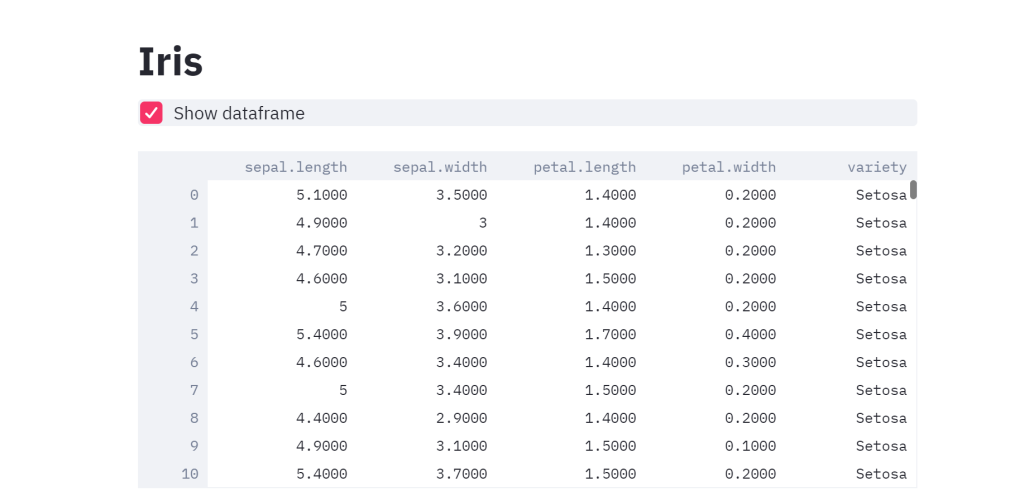As you can see, I decided to show my dataset, but, at any moment, I can hide it by unchecking the box.

Now let’s move towards some visualization tools. Let’s say that I want to scatter plot my data, with the possibility of selecting those features and labels which I’m interested in.

``````species = st.multiselect('Show iris per variety?', df['variety'].unique())
col1 = st.selectbox('Which feature on x?', df.columns[0:4])
col2 = st.selectbox('Which feature on y?', df.columns[0:4])new_df = df[(df['variety'].isin(species))]
st.write(new_df)
fig = px.scatter(new_df, x =col1,y=col2, color='variety')st.plotly_chart(fig)``````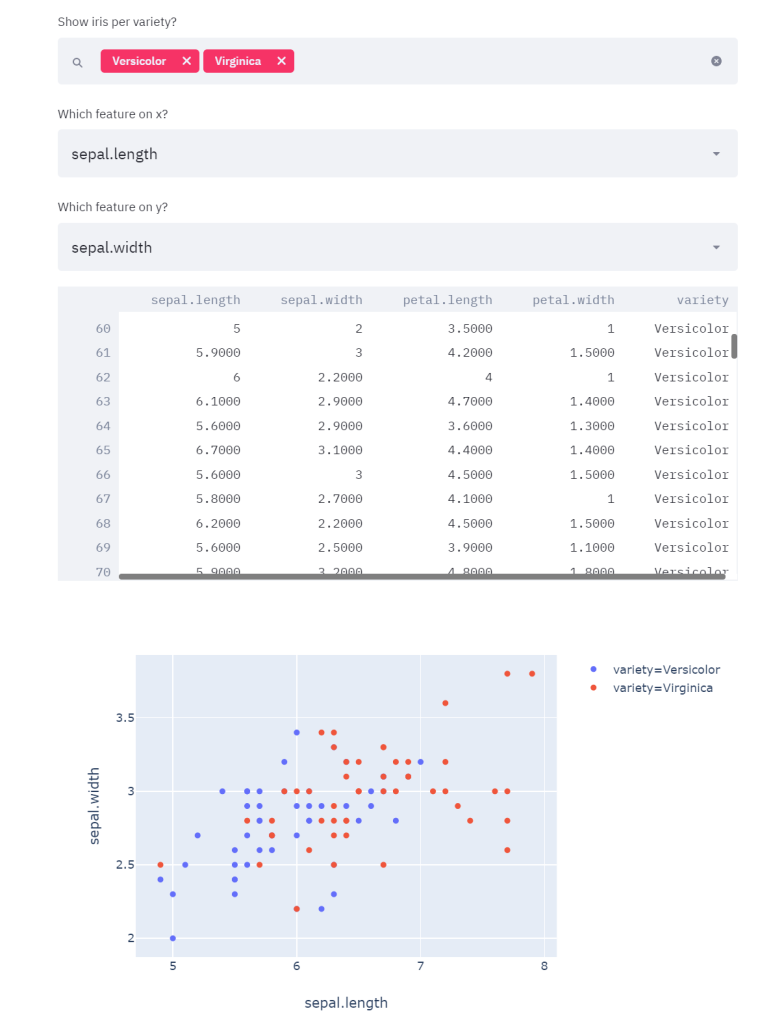As you can see, in the example I selected as species Versicolor and Virginica, as features sepal length and sepal width, but I’m able to change them at any moment and have a real-time updating of all my graphs.

Now I want to add, with the same logic, a histogram that shows the distribution of any feature. Furthermore, I want to have the possibility of plotting the 3 conditional distributions of each feature, with respect to the variety chosen previously. Hence:

``````feature = st.selectbox('Which feature?', df.columns[0:4])
# Filter dataframe
new_df2 = df[(df['variety'].isin(species))][feature]
fig2 = px.histogram(new_df, x=feature, color="variety", marginal="rug")
st.plotly_chart(fig2)``````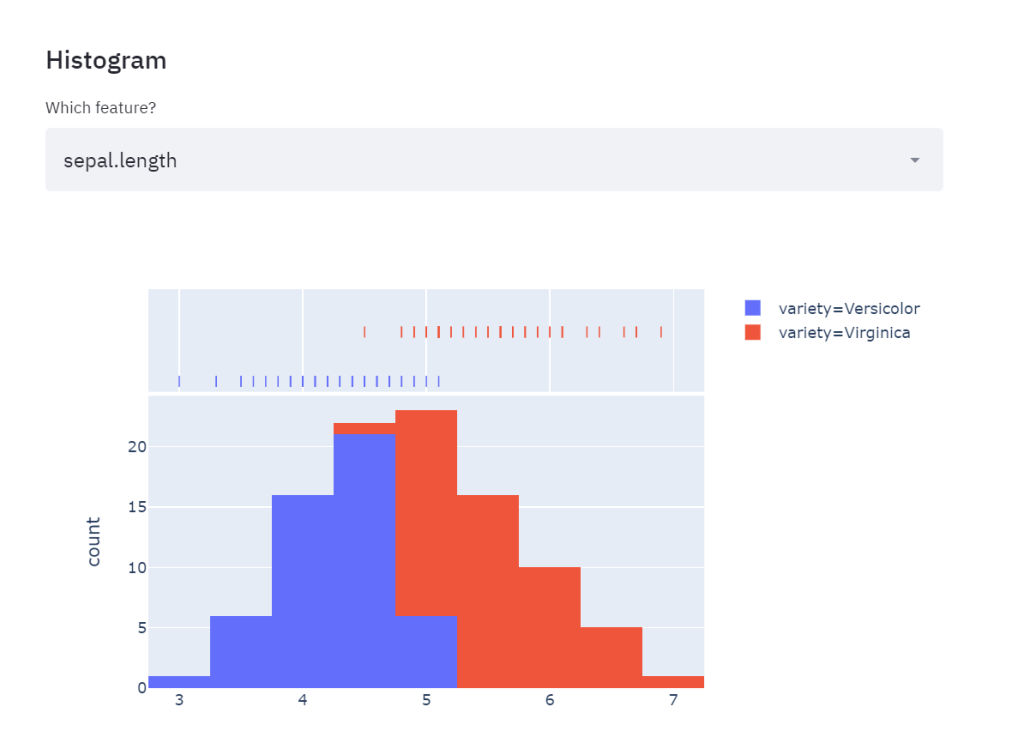The two species are the same as those I picked above and, again, I can change them anytime I want.

Now let’s move to the final part, which is, training real-time ML algorithms and letting the user decide which one to apply. For this purpose, I’m going to set a choice between Support Vector Machine and Decision Tree, both classification algorithms. For each of them, I will ask my app to print the accuracy (number of correctly classified/total number of observations) and the confusion matrix:

``````from sklearn.model_selection import train_test_split
from sklearn.tree import DecisionTreeClassifier
from sklearn.metrics import confusion_matrix
from sklearn.svm import SVCfeatures= df[['sepal.length', 'sepal.width', 'petal.length', 'petal.width']].values
labels = df['variety'].valuesX_train,X_test, y_train, y_test = train_test_split(features, labels, train_size=0.7, random_state=1)alg = ['Decision Tree', 'Support Vector Machine']
classifier = st.selectbox('Which algorithm?', alg)
if classifier=='Decision Tree':
dtc = DecisionTreeClassifier()
dtc.fit(X_train, y_train)
acc = dtc.score(X_test, y_test)
st.write('Accuracy: ', acc)
pred_dtc = dtc.predict(X_test)
cm_dtc=confusion_matrix(y_test,pred_dtc)
st.write('Confusion matrix: ', cm_dtc)elif classifier == 'Support Vector Machine':
svm=SVC()
svm.fit(X_train, y_train)
acc = svm.score(X_test, y_test)
st.write('Accuracy: ', acc)
pred_svm = svm.predict(X_test)
cm=confusion_matrix(y_test,pred_svm)
st.write('Confusion matrix: ', cm)``````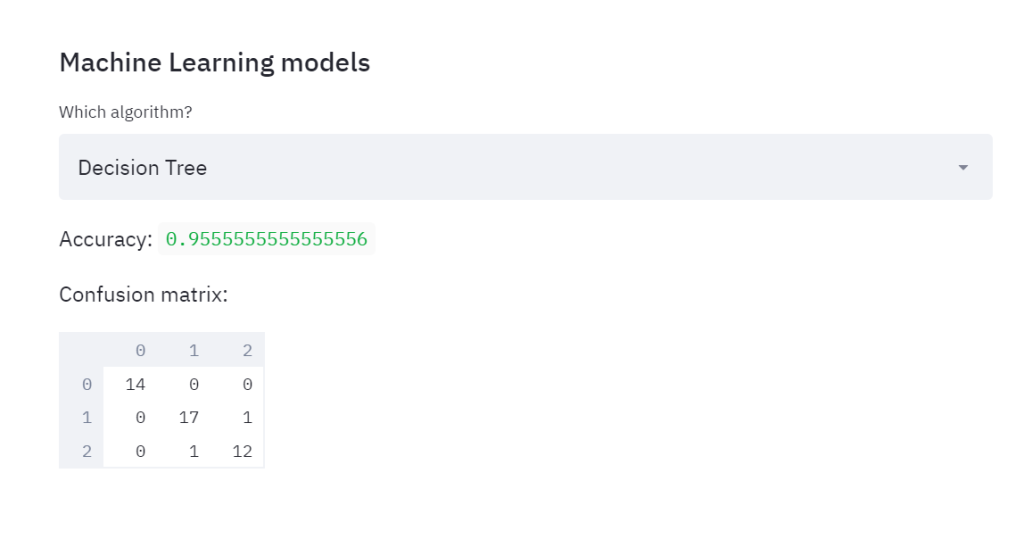Then, if I choose SVM: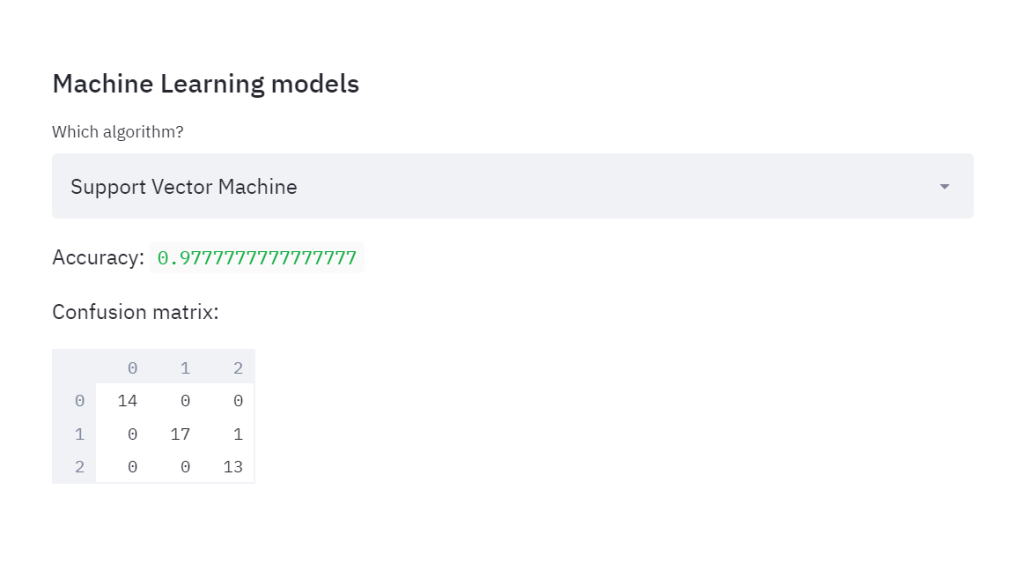So we were able to instantly compare the performances of two classifiers, in a way that is very user-friendly.

Streamlit is a very powerful tool especially if you want to provide an interactive way to understand your analysis’ results: it allows real-time visualization of your data, with the possibility of filtering them, and it allows for meaningful representations.

Here I showed you the very basic implementations you can reach with Streamlit, hence if you want to dive deeper into this tool, I recommend you the further readings among the references.

References:

## What is Supervised Machine Learning

What is neuron analysis of a machine? Learn machine learning by designing Robotics algorithm. Click here for best machine learning course models with AI

## Python For Machine Learning | Machine Learning With Python

Python For Machine Learning | Machine Learning With Python

## Python For Machine Learning | Machine Learning With Python

Python For Machine Learning | Machine Learning With Python, you will be working on an end-to-end case study to understand different stages in the Machine Learning (ML) life cycle. This will deal with 'data manipulation' with pandas and 'data visualization' with seaborn. After this an ML model will be built on the dataset to get predictions. You will learn about the basics of scikit-learn library to implement the machine learning algorithm.

## Python for Machine Learning | Machine Learning with Python

Python for Machine Learning | Machine Learning with Python, you'll be working on an end-to-end case study to understand different stages in the ML life cycle. This will deal with 'data manipulation' with pandas and 'data visualization' with seaborn. After this, an ML model will be built on the dataset to get predictions. You will learn about the basics of the sci-kit-learn library to implement the machine learning algorithm.

## Python For Machine Learning | Machine Learning With Python

🔥 Get the pdf of this course: https://glacad.me/GetPDF_PythonML 🔥 Great Learning brings you this live session on 'Python for Machine Learning'. In this sessi...